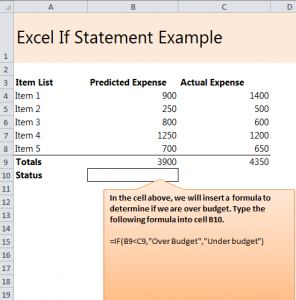# How to do an IF Statement in Excel

7
AprLearn how to do an IF Statement in Excel with our easy guide. An IF statement is when you enter a formula into Excel that will tell you the result of two entries. For instance, IF a number is bigger than another, THEN the outcome is “bigger number”.

How can I use the IF formula in Excel sheets?

• Use the formula to discover what the outcome of a long math problem is in comparison to another.
• See what category has a better status than the other.
• Easily and quickly comparing amounts between each other.

Steps to creating an IF formula:

1. Open the spreadsheet and view the three columns: the items, the predicted expense, and the actual expense. The expense columns are what is important here.
2. The “Totals” at the bottom are what you will derive the IF references from. B9 and C9.
3. In the “Status” bar, type in: =IF(B9<C9,”Over Budget”,”Under Budget”)
4. B9 is less than C9, which indicates that C9 is established as Over Budget.
5. Once the status bar is filled in, your result will appear.